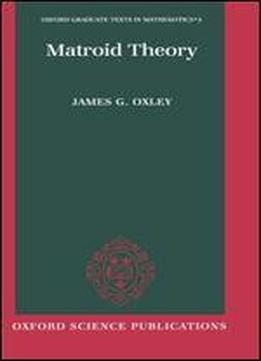# Matroid Theory by J. G. Oxley / 1992 / English / DjVu

What is the essence of the similarity between forests in a graph and linearly independent sets of columns in a matrix? Why does the greedy algorithm produce a spanning tree of minimum weight in a connected graph? Is it possible to test in polynomial time whether a matrix is totally unimodular?These questions form the basis of Matroid theory. The study of matroids is a branch of discrete mathematics with basic links to graphs, lattices, codes, transversals, and projective geometries. Matroids are of fundamental importance in combinatorial optimization and their applications extend into electrical engineering and statics. This book falls into two parts: the first provides a comprehensive introduction to the basics of matroid theory, while the second treats more advanced topics. The book contains over five hundred exercises and includes, for the first time in one place, short proofs of all but one of the majortheorems in the subject. The final chapter lists sixty unsolved problems and describes progress towards their solutions.

views: 149